JAVA, HTML5 & Chemistry Site

Other Writing

Last Update
07-Jan-2015

### Properties Estimation: Estimation of density and volumetric properties

2011.6.15

Lecture note of Dr. Hiroshi Yamamoto

## <!-- document.write("<p>Check the browser <BR>"); var userAgent = window.navigator.userAgent.toLowerCase(); var appVersion = window.navigator.appVersion.toLowerCase(); var canrun=0; if (userAgent.indexOf("msie") > -1) { if (appVersion.indexOf("msie 6.0") > -1) { document.write("IE 6.0 does not support HTML5. Please use Chrome, Safari or FireFox. <BR>"); } else if (appVersion.indexOf("msie 7.0") > -1) { document.write("IE 7.0 does not support HTML5. Please use Chrome, Safari or FireFox. <BR>"); } else if (appVersion.indexOf("msie 8.0") > -1) { document.write("IE 8.0 does not support HTML5. Please use Chrome, Safari or FireFox. <BR>"); } else if (appVersion.indexOf("msie 9.0") > -1) { document.write("IE9 may run this program but out of support. Please use Chrome, Safari or FireFox. <BR>"); } else { document.write("Unkown Browser. Please use Chrome, Safari or FireFox. <BR>"); } } else if (userAgent.indexOf("firefox") > -1) { canrun=1; } else if (userAgent.indexOf("opera") > -1) { canrun=1; } else if (userAgent.indexOf("chrome") > -1) { canrun=1; } else if (userAgent.indexOf("safari") > -1) { canrun=1; } else { document.write("Unkown Browser. Please use Chrome, Safari or FireFox. <BR>"); } if(canrun==1){ document.write("HTML5 will run with this browser. <BR>"); } // -->

Pirika neural network method (JAVA version, NN method, 2004.11.14)
Yen-Woods method (JAVA version, CST method 2004.11.14)
Volume (JAVA version, group additivity method, 2004.11.14)
Volume-Surface (JAVA version, NN method, 2004.11.14)
Volume-Surface (HTML5 version, Test program for YMB, 2011.5.16)
YMB simulator (HTML5 version 2011.6.10, need pass code to use full function）

Newest version is implemented into HSPiP ver.4(Y-Predict). If the corporate visitor want to use full version, please buy HSPiP. The Abstract of HSPiP (2013.1.18)

## Definition:

Density, ratio of the mass of a substance to its volume, expressed, for example, in units of grams per cubic centimeter or pounds per cubic foot. The density of a pure substance varies little from sample to sample and is often considered a characteristic property of the substance. Most substances undergo expansion when heated and therefore have lower densities at higher temperatures. Many substances, especially gases, can be compressed into a smaller volume by increasing the pressure acting on them. For these reasons, the temperature and pressure at which the density of a substance is measured are usually specified. The density of a gas is often converted mathematically to what it would be at a standard temperature and pressure (STP). Water is unusual in that it expands, and thus decreases in density, as it is cooled below 3.98'C (its temperature of maximum density).

## Estimation Method:

There are 2 types of properties that we want to handle in chemistry area. One is the properties that increase/decrease as the molecular size become larger. Boiling point, Critical temperature, heat of vaporization and heat of formation are such properties. This type of properties are suitable for building prediction scheme with Group Contribution method. The other type of properties are independent with molecular size such as density, solubility parameter and refractive index. These properties can not use group contribution method to predict. But in real, there are many properties estimation schemes for these second type of properties. In that case, we need to know the population of that scheme based. But almost all case, it is not opened.

If we apply group contribution method, we need to choose the properties that
will increase/decrease as to increase/decrease of the functional groups. For example, the density case, density is defined as molecular weight divided by molecular volume. So if we can estimate molecular volume with group contribution method, we can get density very easily. YNU-simulator use 167 functional groups and already determined the factor for molecular volume to each functional groups. I validated the accuracy with database compounds and get result like below. The population is 6461 compounds.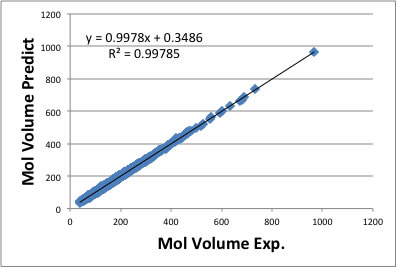To build this QSPR model, I had trouble with the experimental database. Some of the data are not liquid data but solid data. And many melting point data are missing, so I can not find out the density data is solid or liquid. I need to check carefully to remove such data from parent population.

Even though I could not determine all of the factors for 167 functional groups. So I used some software(WinMostar) to calculate molecular volume and surface area. At first , I optimized structure with MOPAC and get these information.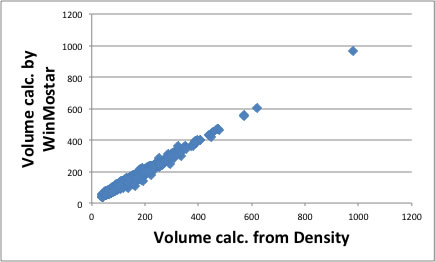These theoretical calculation, the molecule is put in vacuum at 0 Kelvin. In real case, the molecule packing each other and calculated theoretical volume and volume from density is not completely identical. I show the correlation of these relationship.

I already showed the usefulness of volume as fixing parameter for predicting critical temperature and critical pressure. Please refer to that articles.

The density decrease as temperature increase. I show the example of acetone.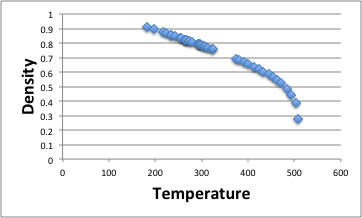The rightest point is critical density and critical temperature. As you see, around critical point, density is changing dramatically. But room temperature to boiling point (329.44k) density decrease almost lineally. Roughly speaking, almost all organic compounds, thermal expansion coefficient is -0.001g/cc for 1℃.
(You can not use this coefficient around critical point.)

There are several methods for estimating pure liquid specific volumes or densities.

• Hankinson Brobst Thomson ( HBT ) method
• Modified Rackett method
• Bhirud's method
• Yen-Woods method

To use these method, critical temperature (Tc), acentric factor, critical pressure (Pc), critical volume (Vc) data are needed.

The most popular method for density estimation is Yen-Woods method. This method is one of the Corresponding state theory (CST) and estimate density with following scheme.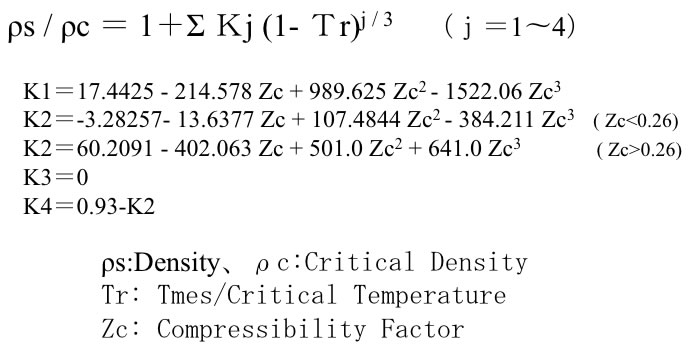With using this scheme, I validated the data listed in ” The properties of Gases & Liquids". The accuracy is very low.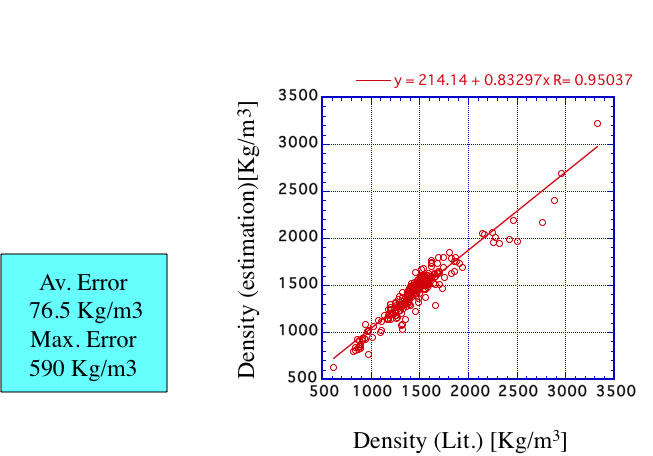Roughly speaking, it is not so bad. But when I calculate Zc (compressive factor), I need Critical temperature(Tc) and Pressure(Pc) , and also need Critical Density.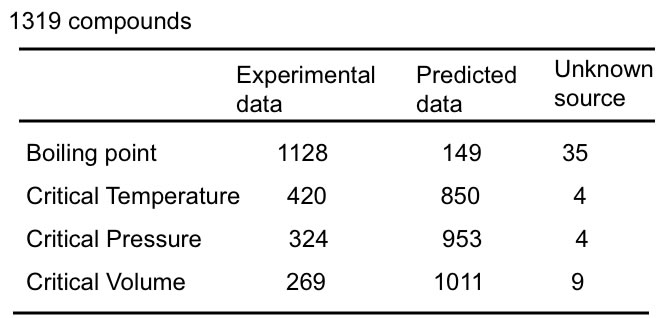For calculating Critical Density, I need Critical Volume(Vc) but experimental Vc is so limited. There is Vc estimation scheme like Joback Method, but it is very hard to expand new compounds like halogenated compounds.

Such compounds that are measured critical properties, are already measured the temperature dependency of density and can utilize from database. So I build QSPR scheme to predict the temperature dependency of density from molecular volume data at room temperature.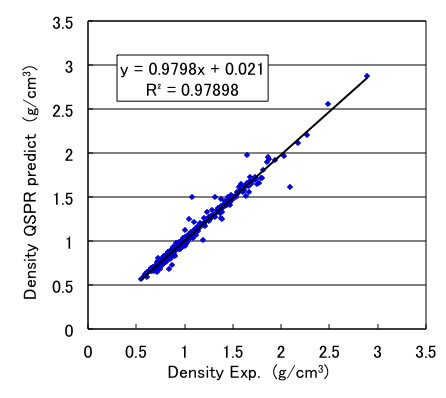Once I build such scheme, I can calculate the density at any temperature. I show the example with Acetone. From only chemical structure, I can estimate temperature dependency of density of liquids.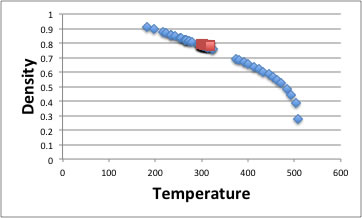This properties is very important to predict other temperature dependent properties such as thermal conductivity or viscosity.

I will explain about molecular surface area here. Unfortunately, we can not get experimental molecular surface area. So I calculated MOPAC and got optimized structure then calculate surface area for 2560 compounds. Then I determined group contribution factors for 167 functional groups.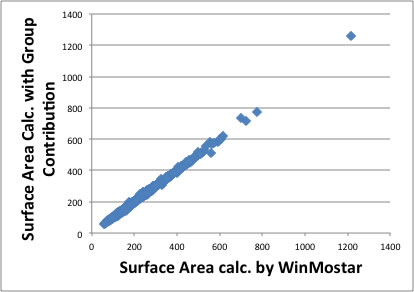I show the result of this calculation, the molecular size become lager, the error also become larger, but the accuracy is I think enough.

I will also explain Ovality here. If the molecular surface area (A) and molecular volume (V) then Ovality is defined with following scheme.
Ovality=A/(4*pi*((3*V)/4*pi)^(2/3))
What does this scheme means? If you have one molecule, the minimum surface area is achieved when the molecule packing complete sphere. At that time Volume =(4/3)*pi*r*r*r.
So, radius can calculate ｒ=( (3*V)/4) ^(1/3).
The surface area = 4*pi*r*r = 4*pi*( (3*V)/4) ^(2/3)
And real surface area A divided by 4*pi*( (3*V)/4) ^(2/3) is ovality. I show you the picture of this relation.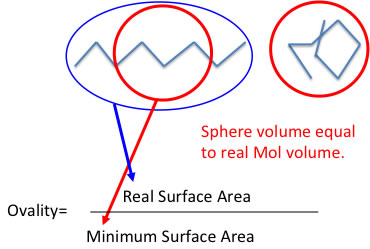The real molecule will not always stretch completely. The molecule that have the large Ovality can stretch or shrink. Some properties are different from this state like solubility. But like CCl4( Ovality equals to 1.0) it can not stretch or shrink. This factor is very useful when we think about viscosity or some other properties.

This Ovality can calculate from the molecular orbital handling software like WinMostar.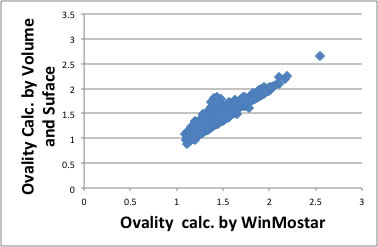But if I use calculated Volume and Surface Area with group contribution method, the error become very large. The reason of this error is come from loss of connectivity information for functional groups. We would better calculate from 3D structure.